Courses

# Vector Calculus - 2

## 20 Questions MCQ Test Topic-wise Tests & Solved Examples for IIT JAM Mathematics | Vector Calculus - 2

Description
This mock test of Vector Calculus - 2 for IIT JAM helps you for every IIT JAM entrance exam. This contains 20 Multiple Choice Questions for IIT JAM Vector Calculus - 2 (mcq) to study with solutions a complete question bank. The solved questions answers in this Vector Calculus - 2 quiz give you a good mix of easy questions and tough questions. IIT JAM students definitely take this Vector Calculus - 2 exercise for a better result in the exam. You can find other Vector Calculus - 2 extra questions, long questions & short questions for IIT JAM on EduRev as well by searching above.
QUESTION: 1

###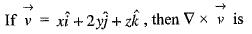Solution: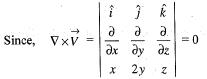QUESTION: 2

###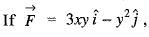then the value of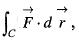where C is the curve in the XY-plane, y = 2x2 from (0,0) to (1,2) is

Solution:

Explanation : y = 2x2

dy/dx = 4x

So F. dr

∫(x = 0 to 1) [3x(2x2)dx . (2x2)2 d(2x2)]

∫(x = 0 to 1) (6x2 - 16x5)dx

= -7/6

QUESTION: 3

### Value of the integral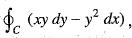where C is the square cut from the first quadrant by the lines x = 1 and y = 1 will be (use Green’s theorem to change the line integral into double integral)

Solution:

We know that in Green’s theorem,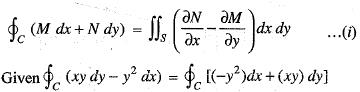On comparing, we get M = -y2 and N = xy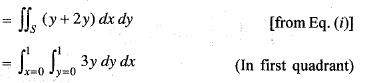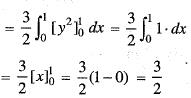QUESTION: 4

The divergence of the vector field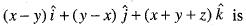Solution: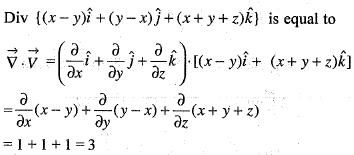QUESTION: 5

The directional derivative of f(x, y, z) = x2 + y2 + z2 at the point (1, 1, 1) in the direction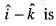Solution:

We have,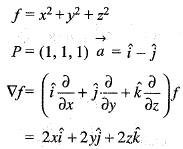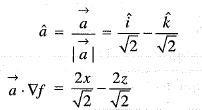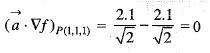Thus, directional derivative of f in the direction of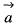the point P(1, 1, 1),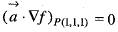QUESTION: 6

The unit normal vector to the surface of the sphere x2 + y2 + z2 = 1 at the point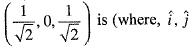and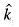are unit normal vectors in the Cartesian coordinate system)

Solution: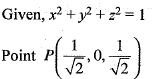Problem is to find unit normal vector to the surface of the sphere
f = x2 + y2 + z2 - 1 = 0
We know unit normal vector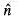is given by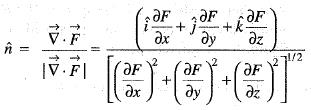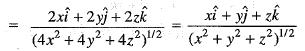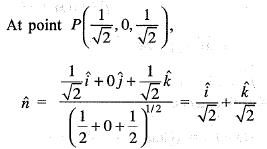QUESTION: 7

If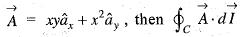over the path shown in the figure is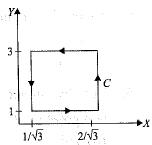Solution: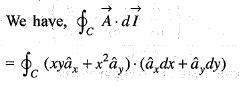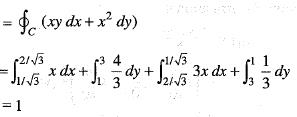QUESTION: 8

Apply Green’s theorem the value of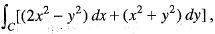where C is the boundary of the area enclosed by the X-axis and the upper half of the circle x2 + y2 = a2 is

Solution: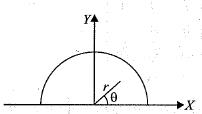Since, From Green’s theorem, we have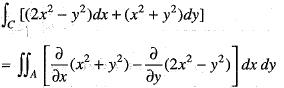(A is the region of the figure)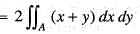On changing in polar coordinates, we get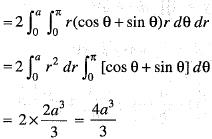QUESTION: 9

A fluid element has a velocity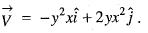The motion at (x, y) =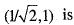Solution: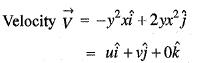or u = - y2x, v = 2yx2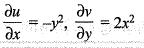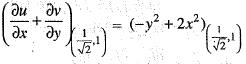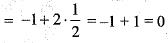In two-dim ensional flow, equation of continuity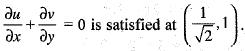Fluid is incompressible at this point.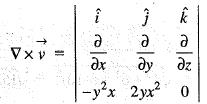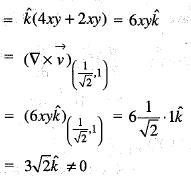Fluid flow is rotational.
Thus, fluid flow at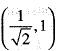is rotational and incompressible.

QUESTION: 10

Unit vectors in X and Z-directions are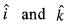respectively. Which one of the following is the directional derivative of the function F(x, z) = In (x2 + z2) at the point P(4, 0), in the direction of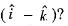Solution:

Given, F(x, y) = In(x2 + z2) = log (y + z2)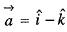Coordinate of point p is (4, 0).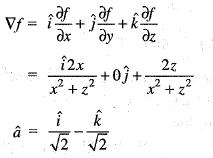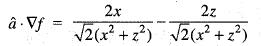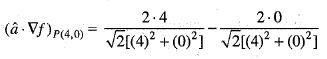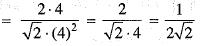or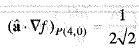QUESTION: 11

For a scalar function f(x, y, z) = x2 + 3y2 + 2z2, the gradient at the point P(1, 2, -1) is

Solution: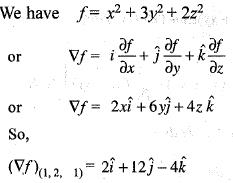QUESTION: 12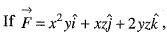then the value of div Curl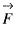is

Solution: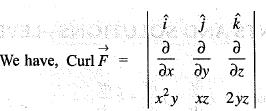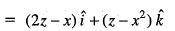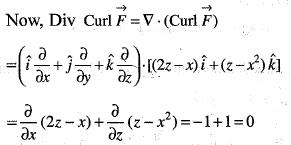QUESTION: 13

The value of α for which the following three vectors are coplanar is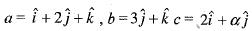Solution:

Given,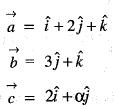Vectors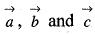are coplanar, if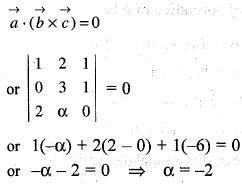QUESTION: 14

Apply Green’ s theorem the value of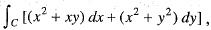where C is the square formed by the lines y = ±1, x = ±1 is

Solution:

Since, On comparing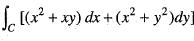with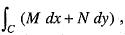we get M = x2 + xy and
N = x2 + y2
So, from Green’s theorem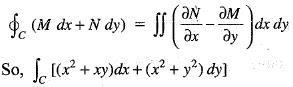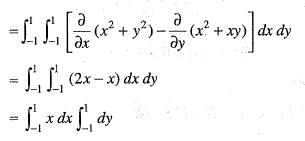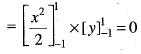QUESTION: 15

The divergence of the vector field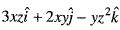at a point (1, 1, 1) is equal to

Solution:

Since, We have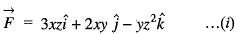On comparing Eq. (r) with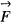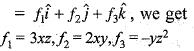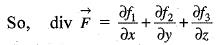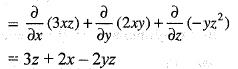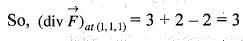QUESTION: 16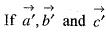is the reciprocal system to the vectors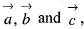then the value of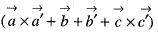Solution:
QUESTION: 17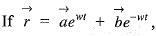where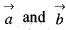are constant vectors then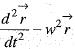is equal to

Solution:
QUESTION: 18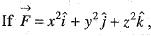, then the value of div curl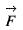is

Solution:
QUESTION: 19

The value of div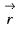will be

Solution:

If P (x,y,z) is a variable point in a three- dimensional space, O the origin, i, j and k the unit vectors along the x-axis, y-axis and z-axis respectively, then the vector OP given by x i + y j +z k is called the position vector of the point P and is denoted by r.

Divergence of any vector f = f1i+ f2j +f3k denoted by div f is defined as the scalar ( delta f1)/(delta x) + (delta f2)/(delta y) + (delta f3)/(delta z)

where the delta s denote partial derivatives.

Using this definition we find that div r = delta (x)/ delta x +delta (y)/delta y + delta (z)/ delta z = 1 + 1 +1 = 3.

Hence, divergence of a position vector = div r = 3.

QUESTION: 20

Divergence operators is defined for

Solution: